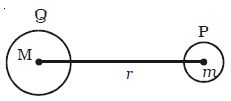Physics

# Explain Gravitational Field

Two masses separated by a distance exert gravitational forces on one another. This is called action-at-a-distance. They interact even though they are not in contact. This interaction can also be explained with the field concept. A particle or a body placed at a point modifies a space around it which is called gravitational field. When another particle is brought in this field, it experiences the gravitational force of attraction. The gravitational field is defined as the space around a mass in which it can exert the gravitational force on other mass.Gravitational field intensity: Gravitational field intensity or strength at a point is defined as the force experienced by a unit mass placed at that point It is denoted by E. It is a vector quantity. Its unit is N kg-1

Consider a body of mass M placed at a point Q and another body of mass M placed at P at a distance r from Q.

The mass M develops a field E at P and this field exerts a force F = mE.

The gravitational force of attraction between the masses m and M is F = GMm/R2

The gravitational field intensity at P is E = F/m

So, E = GM/R2

Gravitational field intensity is the measure of the gravitational field.